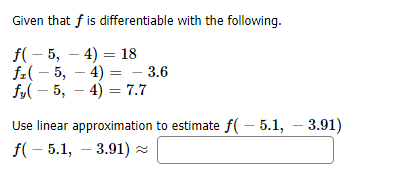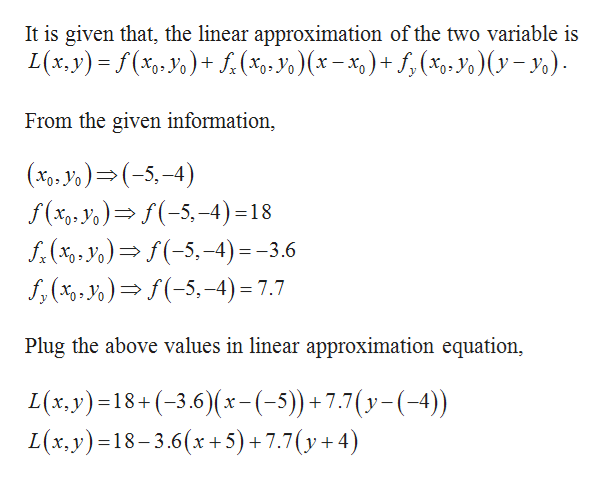# Given that fis differentiable with the followingf(5, 4) 18f5, 4)- 3.6fy5, 47.7Use linear approximation to estimate f( 5.1, 3.91)f(5.1, 3.91)

Question
1 viewshelp_outlineImage TranscriptioncloseGiven that fis differentiable with the following f(5, 4) 18 f5, 4) - 3.6 fy5, 47.7 Use linear approximation to estimate f( 5.1, 3.91) f(5.1, 3.91) fullscreen
check_circle

Step 1help_outlineImage TranscriptioncloseIt is given that, the linear approximation of the two variable is L(x, y) f(xp. y)+(%0-o)(x-x,)+ f(% o)(yy From the given information, , Jo)(-5-4) f(xp, yo) (-5-4) =18 f.(%-)-5,-4)=-3.6 f,(4)-5,-4) = 7.7 Plug the above values in linear approximation equation, L(x. y)18+(-3.6)(x-(-5) + 7.7 (y- (-4)) L(x.y) 18-3.6(x+5) +7.7(y + 4) = fullscreen

### Want to see the full answer?

See Solution

#### Want to see this answer and more?

Solutions are written by subject experts who are available 24/7. Questions are typically answered within 1 hour.*

See Solution
*Response times may vary by subject and question.
Tagged in

### Other